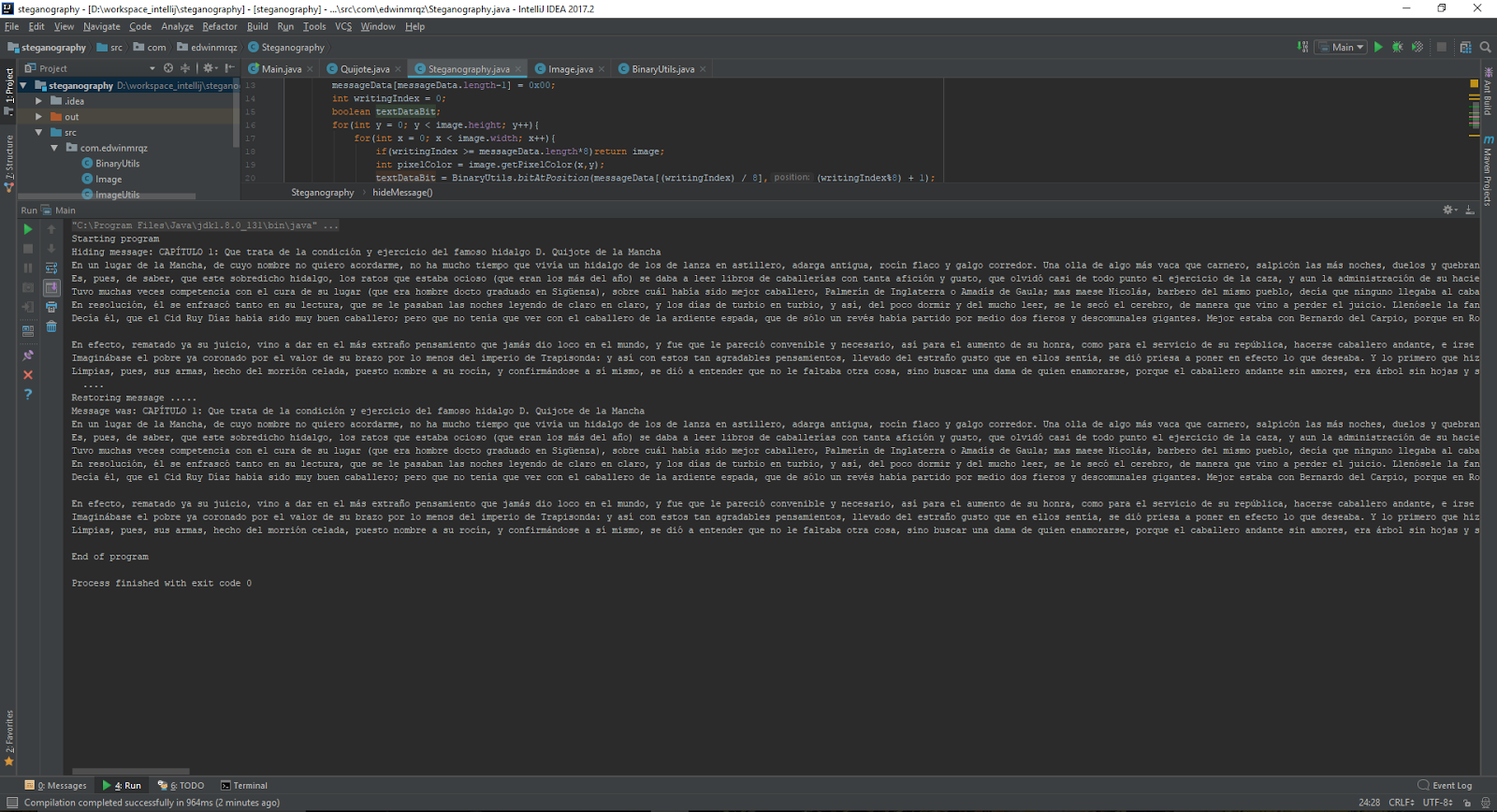# Steganography. Hiding messages in Pictures.

## (Things to do with a computer and some free time)

What about hiding a text in an image?
How different will it look like?
The idea is simple. Let's separate the task in steps.
1.) Get an image, and this incredible (CC0)image works for this project.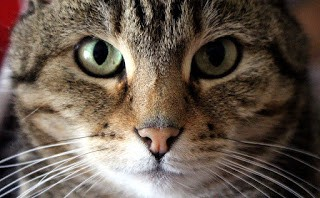Cat . link: https://pixabay.com/en/cat-face-eyes-view-portrait-1605317/

Giving that the tasks is at a bit level, Jpg is not a format that we can use for this purpose, since its a "lossy compression" format,
The solution is simply to convert the image to another format (Png for example.) that doesn't modify its information.

2) Decide the strategy to follow.

There is a lot of options to approach this task, but to keep things simple and to avoid doing noticeable modifications, modifying the less significant bit for every color seem reasonable.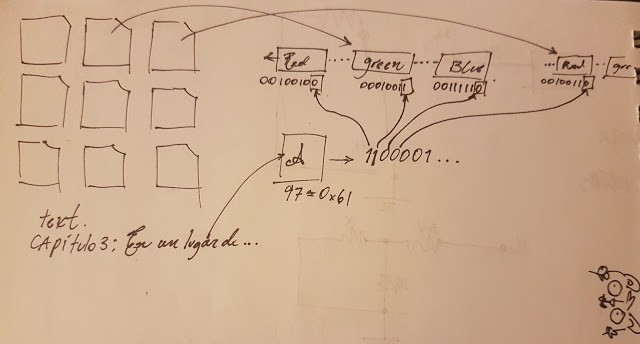The strategy is this: every image is made of hundreds of pixels, of every pixel choose some colors, in this case red, green and blue. of every value take the less significant bit and replace it with a bit on the text.

Now some numbers!

Given that the image has a resolution of 960 x 593 pixels, from which we use 3 bits to represent characters, every character been 8bits.
in summary, we can represent 960*593*3/8 characters. = 213,480

3) Implement the solution.
For this particular case java.awt.image is a good option to use.

``````
private BufferedImage image;

``````

Internally the information about the colors is coded into an integer (int). so it is necessary to use operations of the kind:

``````
public int aRGBToColor(int a, int r, int g, int b){
return (a<<24)|(r << 16)|(g<<8)|b; }

``````

where a represents the alpha color, r red, g green, and b blue. We also need functions to extract the information from the integer. for example:

``````
public static int getRedFromColor(int color){
return color<<8>>>24;}

``````

the coding is nothing more than using this functions to store the desire text bit into the color bit, and for decoding to read this bit.

Result:

The difference between the images can be seen in the next section: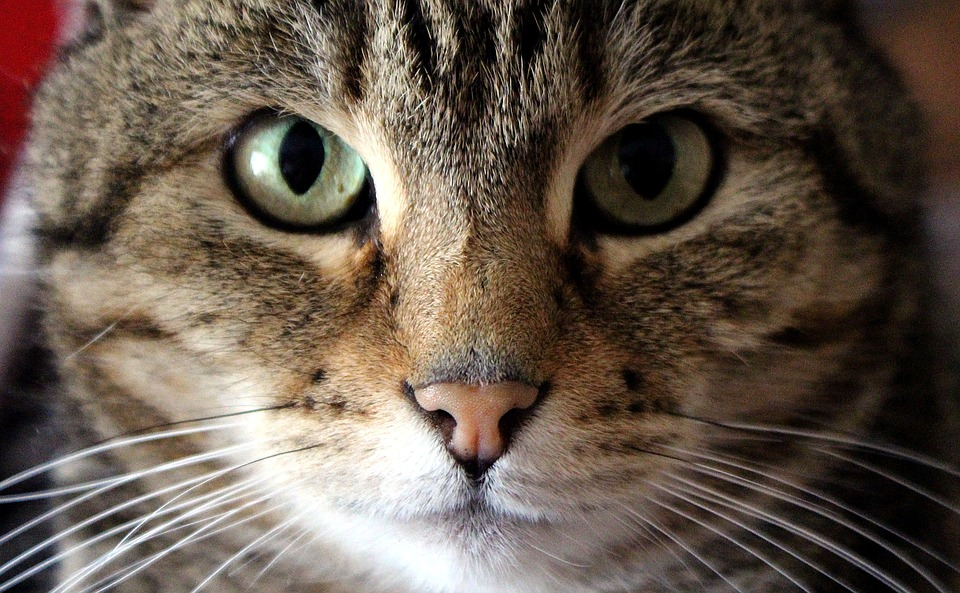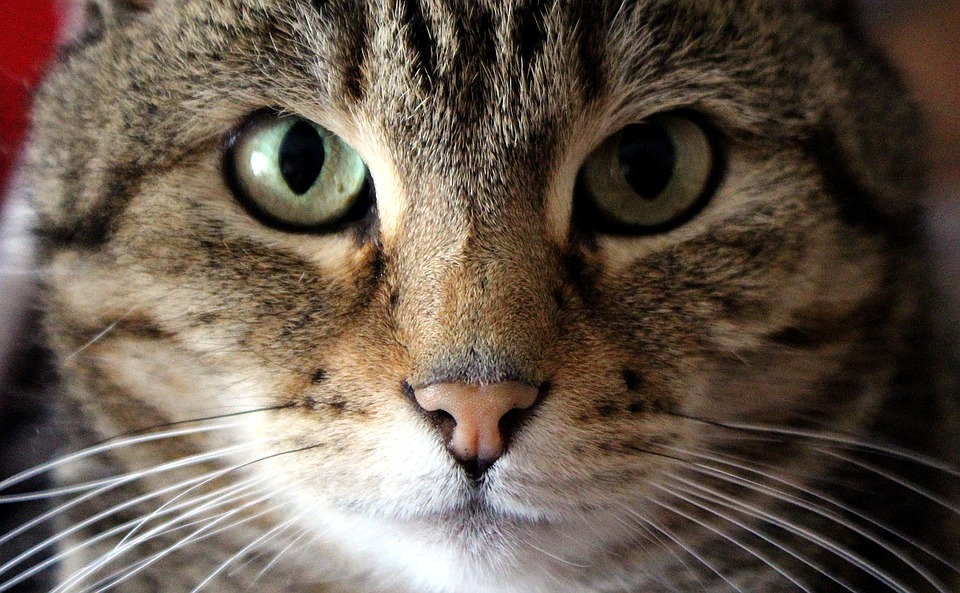The image on top is the original image, the image on the bottom contains the first chapter of Don quijote of La mancha.